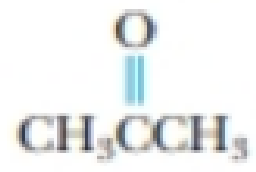Chapter 23, Problem 58PS

Chapter
Section
Textbook Problem

Identify the class of each of the following compounds, and give the systematic name for each:(a)(b)(c)(a)

Interpretation Introduction

Interpretation: The name of the given compund should be given and the class of the compund has to be identified

Concept introduction:

Ketone: Ketone is a group of organic compound where two aryl or alkyl groups are connected by a carbonyl group. It is represented as RC(=O)R'.

The IUPAC name for the esters is written by replcing the ‘e’ of alkanes from ‘one’.

Explanation

The given compound is,

It belongs to carbonyl compounds as it has R2CO functional group

(b)

Interpretation Introduction

Interpretation: The name of the given compund should be given and the class of the compund has to be identified

Concept introduction:

Ketone: Ketone is a group of organic compound where two aryl or alkyl groups are connected by a carbonyl group. It is represented as RC(=O)R'.

The IUPAC name for the esters is written by replcing the ‘e’ of alkanes from ‘one’.

(c)

Interpretation Introduction

Interpretation: The name of the given compund should be given and the class of the compund has to be identified

Concept introduction:

Aldehyde: One alkyl group and a hydrogen atom are attached to the carbonyl carbon atom of the compound. It is represented as RCHO.

The IUPAC name for the esters is written by replcing the ‘e’ of alkanes from ‘al’.

Still sussing out bartleby?

Check out a sample textbook solution.

See a sample solution

The Solution to Your Study Problems

Bartleby provides explanations to thousands of textbook problems written by our experts, many with advanced degrees!

Get Started